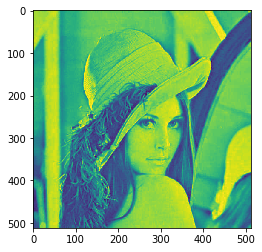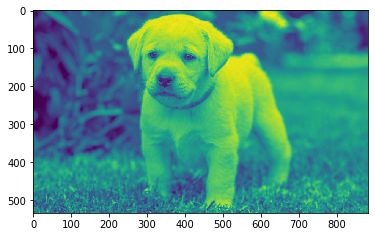# Mahotas – Center of Mass of given Image

• Last Updated : 26 May, 2021

In this article we will see how we can get the center of mass of the image in mahotas. Center of mass” (for binary images) is a bit convoluted way of saying “mean value across each dimension”. In other words – take all x coordinates and average them – and you got x coordinate of your “center of mass”, the same for y.
In this tutorial we will use “lena” image, below is the command to load it.

`mahotas.demos.load('lena')`

Below is the lena image

Attention geek! Strengthen your foundations with the Python Programming Foundation Course and learn the basics.

To begin with, your interview preparations Enhance your Data Structures concepts with the Python DS Course. And to begin with your Machine Learning Journey, join the Machine Learning - Basic Level CourseIn order to do this we will use mahotas.center_of_mass method
Syntax : mahotas.center_of_mass(img)
Argument : It takes image object as argument
Return : It returns center of mass co-ordinates

Note : Input image should be filtered or should be loaded as grey
In order to filter the image we will take the image object which is numpy.ndarray and filter it with the help of indexing, below is the command to do this

`image = image[:, :, 0]`

Below is the implementation

## Python3

 `# importing required libraries``# importing required libraries``import` `mahotas``import` `mahotas.demos``from` `pylab ``import` `gray, imshow, show``import` `numpy as np`` ` `# loading image``img ``=` `mahotas.demos.load(``'lena'``)` `# grey image``g ``=` `img[:, :, ``1``]` `# multiplying grey image values``g ``=` `g ``*` `100`` ` `# filtering image``img ``=` `img.``max``(``2``)`` ` `# showing image``imshow(img)``show()` `# getting center of mass``center ``=` `mahotas.center_of_mass(img)`` ` `# printing center of mass co-ordinate``print``(``"Center of Mass : "` `+` `str``(center))`

Output :`Center of Mass : [246.64854256 259.45157125]`

Another example

## Python3

 `# importing required libraries``import` `mahotas``import` `numpy as np``from` `pylab ``import` `gray, imshow, show``import` `os`` ` `# loading image``img ``=` `mahotas.imread(``'dog_image.png'``)` ` ` `# filtering image``img ``=` `img[:, :, ``0``]`` ` `# showing image``imshow(img)``show()` `# getting center of mass``center ``=` `mahotas.center_of_mass(img)`` ` `# printing center of mass co-ordinate``print``(``"Center of Mass : "` `+` `str``(center))`

Output :`Center of Mass : [265.35619268 482.66701402]`

My Personal Notes arrow_drop_up